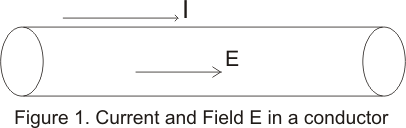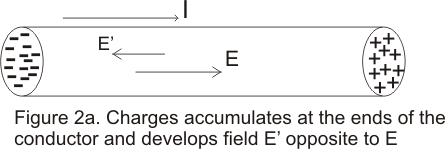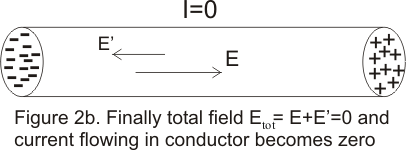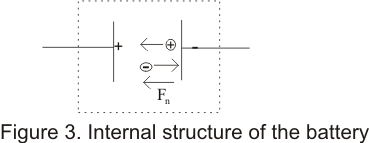# Electromotive Force|EMF

## (1) Introduction

• In previous chapter we have already studied about electric current and resistance.
• We know that a force must be applied on free charges of a conductor in order to maintain a continous current in the conductor.
• Here a question arises how can we maintain this force in order to maintain a continous flow of current. You will find answer to this question while studying this chapter.
• In this chapter we will learn about ElectroMotive Force(emf) and sources of emf ( responsible for driving charge round the closed circuit). We'll also learn about electric circuits and measurements.

## (2) ElectroMotive Force(emf)

• Consider a conductor lying in presence of electric field as shown below in the figure such that an electric field exists inside the conductor.• We know that when electric field exists in a conductur electric current begins to flow inside the conductor. Now a question arises what happens to the
charge carriers when they reach the ends of the conductor and would this current remains constant with the passage of time.
• We can easily conclude that for an open ended conductor as shown in the figure , charges would accumulate at the ends of the conductor resulting a change in electric field with the passage of time. Due to this electric current would not remain constant and would flow only for a very short interval of time , digrametically shown below in the figure.• Thus, in order to maintain a steady current throughout a conducting path the path must be in the form of a closed loop forming a complete circuit. Even this condition is not sufficient to maintain a steady current in the circuit.
• This is because charge always moves in the direction of decreasing potential and electric field always does a positive work on the charge.
• Now after travelling through a complete circuit when charge returns to a point where it has started, potential at that point must be same as the potential at that point in the begning of the journey but flow of current always involves loss of potential energy.
• Hence we need some external source in the circuit in which maintains a potential difference at its terminals by increasing the potential energyof the electric charge.
• Such a source make charge travel from lower potential to higher potential energy in direction opposit to the electrostatic force trying to push charge from higher potential to lower potential.
• This force that makes charge move from lower potential to higher potential is called electro mative force (EMF).
• The source or device which provide emf in a complete circuit is known as source of EMF and examples of such devices are generator, batteries, thermocuples etc.
• The source of EMF are basically energy converters that convert mechanical, thermal, chemical or any other form of energy into electrical potential energy and transform it into the circuit to which the source of emf is connected.
• Now we know that a source of emf or battery maintains a potential difference between its two terminals as shown in below figure• Generally a battery consist of two terminals one positive and other is negative.
• Some internal force Fn generally non electric in nature is exerted on the charges of the material of the battery. This non-electric force depends on the nature of source of EMF.
• These Force(Fn) drives the positive charges of the material towards P and nigative charges of the material towards Q. This battery force
Fn is directed Q to P.
• Positive charge accumulate on plate Pand negative charge accumulate on plate Q and a potential developes between plates P and Q. Thus an electric field would set up inside the battery from P to Q which excert an electric force on the charge of the material.
• When a steady state is reached, the the electric force and battery force Fn would become equal and opposit
mathematically,
qE=Fn                                (1)
and after a steady state is reached no further accumulation of charhe takes place.
• Workdone by battery force Fn in taking poisitive charge from terminal Q to terminal P would be
W=Fnd
where d is distance between plates P and Q.
• Workdone by force Fn per unit charge is
EMF=W/q=Fnd/q                                (2)
where the quantity E is known as E.M.F. of the battery.
ENF=qEd/q=ED=V                                (3)
where Veq=Ed is the potential difference across the terminals of the battery when nothing is connected externally between P and (i.e. when circuit is open)